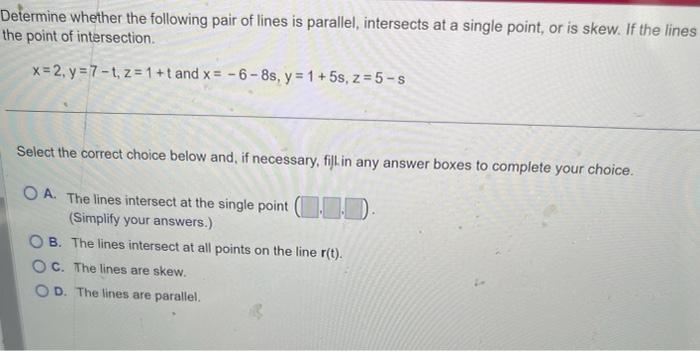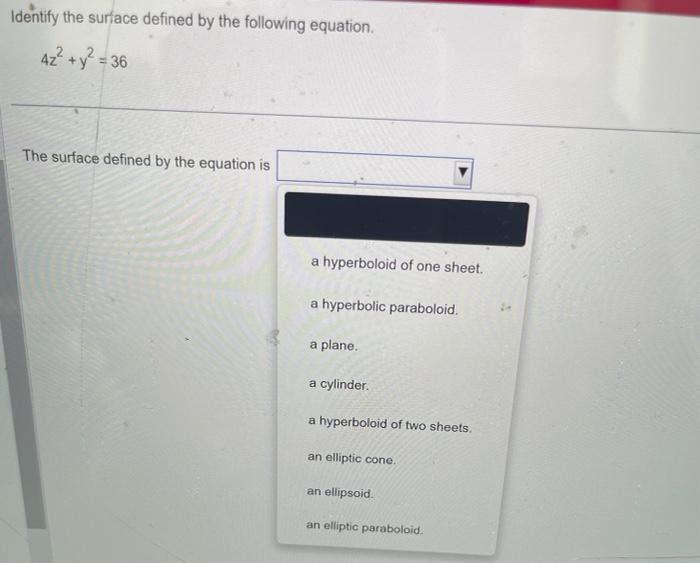# (Solved): please help Determine whether the following pair of lines is parallel, intersects at a single point, ...Determine whether the following pair of lines is parallel, intersects at a single point, or is skew. If the lines the point of intersection. $x=2, y=7-t, z=1+t \text { and } x=-6-8 s, y=1+5 s, z=5-s$ Select the correct choice below and, if necessary, fill in any answer boxes to complete your choice. A. The lines intersect at the single point (Simplify your answers.) B. The lines intersect at all points on the line $$r(t)$$. C. The lines are skew. D. The lines are parallel. Identify the surface defined by the following equation. $4 z^{2}+y^{2}=36$

We have an Answer from Expert